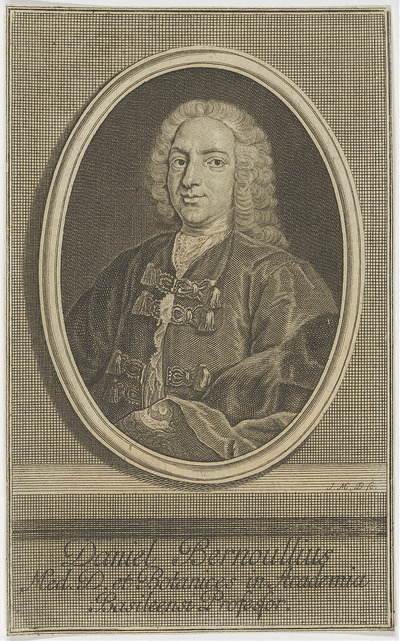## Entrainment: What is it?By definition, entrainment is a form of the verb, entrain, which is fluid that is swept along into an existing moving flow.   Whenever there is a discussion about fluid dynamics, the Bernoulli’s equation generally comes up.  This equation is unique as it relates flow energy with kinetic energy and potential energy.  The formula was mainly linked to incompressible fluids, but under certain conditions, it can be significant for gas flows as well.  I would like to discuss how EXAIR uses the Bernoulli’s equation for entrainment to maximize efficiency within your compressed air system.

This relationship between pressure as compared to flow and velocity came to be known as the Bernoulli’s principle.  “In fluid dynamics, Bernoulli’s principle states that an increase in the speed of fluid occurs simultaneously with a decrease in static pressure or a decrease in the fluids potential energy”1. Bernoulli realized that the sum of kinetic energy, flow energy, and potential energy is a constant during steady flow.  He wrote the equation like this:

Equation 1:

P/r + V2/2 + gz = constant

P – Pressure

r – density

V – velocity

g – gravitational constant

z – height difference

Not to get too technical, but you can see the relationship between the velocity squared and the pressure from the equation above.  Being that this relationship is a constant along the streamline; when the velocity increases; the pressure has to come down.  An example of this is an airplane wing.  When the air velocity increases over the top of the wing, the pressure becomes less.  Thus, lift is created and the airplane flies.

Since we know the criteria to apply the Bernoulli’s equation with compressed air, let’s look at some EXAIR products.  Blowing compressed air to cool, clean, and dry, EXAIR can do it very efficiently as we use the Bernoulli’s principle to entrain the surrounding air.  Remember from the equation above, as the velocity increases, the pressure has to decrease.  When the pressure decreases, the surrounding air will move toward the low pressure.  That low pressure will sweep the ambient air into the air stream; called entrainment.

Compressed air is expensive, but the ambient air is free.  The more ambient air we can entrain, the more efficient the blowing device is.  As an example, we engineer the Super Air Knife to maximize this phenomenon to give an amplification ratio of 40:1. So, for every 1 part of compressed air, the Super Air Knife will bring into the air streamline 40 parts of ambient “free” air.  This makes the Super Air Knife one of the most efficient blowing devices on the market.  By adding mass to the flow stream, it will reduce the compressed air usage, saving you money, and allow for better cooling and a stronger blowing force.  For a drilled pipe, the amplification ratio is generally only two to three times.

We use this principle for many of our products like the Air Amplifiers, Safety Air Guns, Air Nozzles, Air Knives, and Gen4 Static Eliminators. Daniel Bernoulli was able to find a relationship between velocities and pressures, and EXAIR was able to use this to create efficient, safe, and effective compressed air products.  To find out how you can use this advantage to save compressed air in your processes, you can contact an Application Engineer at EXAIR.  We will be happy to help you.

John Ball
Application Engineer
Email: johnball@exair.com